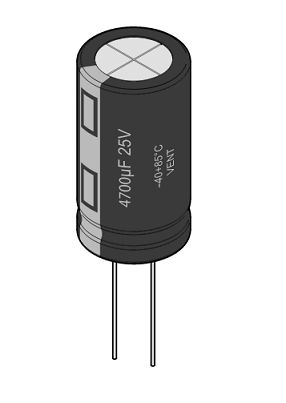# CapacitanceFigure 1. Drawing of a capacitor with the capacitance, 400 microfarads, marked on the side.

Capacitance is the ability of an object (material in a particular geometry) to store an electric charge. Specifically, it is a measure of an isolated conductor's ability to store charge at a given voltage difference. In this sense, an object's capacitance is the ratio between its charge at a particular voltage difference and that voltage difference. Functionally, this leads to capacitance also being a measure of how much energy a capacitor can store.

Often a capacitance is thought of as being the physical property of a capacitor that has two conducting plates close to each other. The capacitance is described mathematically as:

• is the capacitance, measured in farads
• is the charge that is on the positive plate of the capacitor, measured in coulombs
• is the voltage of the conductor, measured in volts

Capacitance is measured in farads (F), with 1 farad representing 1 coulomb per volt. This means that if an isolated conductor had a capacitance of 1 farad and was charged with 1 coulomb, it would have a voltage of 1 volt on its surface. There is also an alternate way to determine the capacitance of a capacitor if its dimensions are known. If the area of the plates of the capacitor can be determined, the capacitance can be calculated from the expression:

• is the capacitance, measured in farads (F)
• is a constant called the permittivity of free space = 8.854188x10-12 F/m 
• is the relative permittivity of the material between the plates
• is the surface area of the plates of the capacitor, measured in square metres (m2)
• is the distance between the plates, measured in metres (m)

The equation shows that the capacitance is influenced by the dimensions of the capacitor.